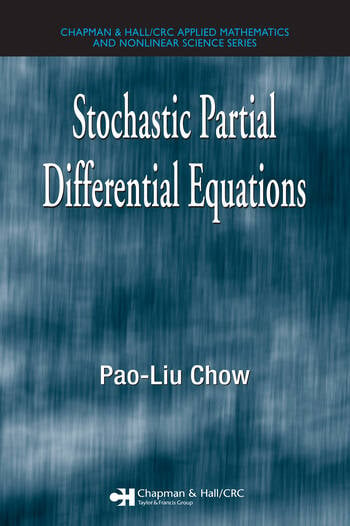Stochastic Partial Differential Equations

1st Edition

Pao-Liu Chow

Chapman and Hall/CRC
Published March 19, 2007
Reference - 281 Pages - 30 B/W Illustrations
ISBN 9781584884439 - CAT# C4436

For Instructors Request Inspection Copy

USD\$110.00

FREE Standard Shipping!

Preview

Summary

As a relatively new area in mathematics, stochastic partial differential equations (PDEs) are still at a tender age and have not yet received much attention in the mathematical community. Filling the void of an introductory text in the field, Stochastic Partial Differential Equations introduces PDEs to students familiar with basic probability theory and Itô's equations, highlighting several computational and analytical techniques.

Without assuming specific knowledge of PDEs, the text includes many challenging problems in stochastic analysis and treats stochastic PDEs in a practical way. The author first brings the subject back to its root in classical concrete problems. He then discusses a unified theory of stochastic evolution equations and describes a few applied problems, including the random vibration of a nonlinear elastic beam and invariant measures for stochastic Navier-Stokes equations. The book concludes by pointing out the connection of stochastic PDEs to infinite-dimensional stochastic analysis.

By thoroughly covering the concepts and applications of stochastic PDEs at an introductory level, this text provides a guide to current research topics and lays the groundwork for further study.

Instructors

We provide complimentary e-inspection copies of primary textbooks to instructors considering our books for course adoption.